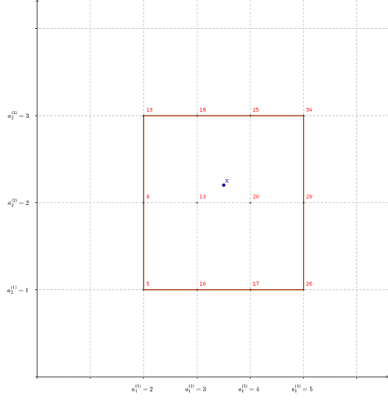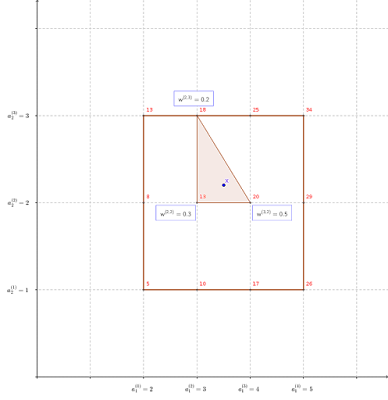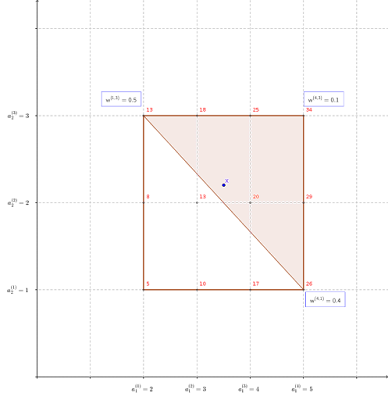## Sunday, March 11, 2018

### Piecewise Linear Approximations in MIP Models

In the past, I've written about piecewise linear approximations of functions of a single variable. (There are too many posts to list here. Just type "piecewise linear" in the blog search box if you want to find them.) Handling piecewise linear approximations of multivariable functions is a bit more intimidating. I'll illustrate one approach here.

To set the stage, assume an optimization problem involving variables $x\in\Re^{n}$, and possibly some other variables $y\in\Re^{m}$. Some or all of the $x$ and $y$ variables may be restricted to integer values. Also assume that the objective function and constraints are all linear, with the exception of one nonlinear function of the $x$ variables that may appear in one or more constraints and/or the objective function. I'll denote that function $f:\Re^{n}\rightarrow\Re$. With that, I'll write our hypothetical problem as
\begin{align*}
\text{min } & g(x,y,z)\\
\text{s.t. } & z=f(x)\\
& (x,y,z)\in D
\end{align*}where $z$ is a new variable that captures the value of $f$, the domain $D$ incorporates all other functional constraints, bounds and integrality restrictions, and the objective function $g$ is linear. So if it were not for the constraint $z=f(x)$, this would be a mixed integer linear program.

I'm also going to assume that we know a priori some hyperrectangle $R\subset\Re^{n}$ containing all feasible values of $x$, say $R=\prod_{i=1}^{n}[L_{i},U_{i}]$. Brace yourself, because the notation is about to get a bit messy. We will create a mesh of discrete points at which $f$ will be evaluated. First, we specify a sequence of what I will call "grid values" for each individual component of $x$. I'll denote the $j$-th grid value for $x_{i}$ by $a_{i}^{(j)}$, where $$L_{i}=a_{i}^{(1)}<a_{i}^{(2)}<\cdots<a_{i}^{(N_{i})}=U_{i}.$$The number of grid values need not be the same for each variable (hence the subscript on $N_{i}$), and the spacing need not be equal. It probably makes sense to sample $f()$ more frequently in regions where its curvature is greater, and less frequently in regions where it is fairly flat.

I'll use the term "mesh points" for the points$$\prod_{i=1}^{n}\left\{ a_{i}^{(1)},\dots,a_{i}^{(N_{i})}\right\}$$formed by combinations of grid values, and $a^{(j)}$ will denote a generic mesh point $(a_{1}^{(j_{1})},\dots,a_{n}^{(j_{n})})$. The superscript $j$ for $w$ is a vector $(j_{1},\dots,j_{n})$ whose domain I will denote $J$. Now we can get down to the piecewise linear approximation. We will write $x$ as a convex combination of the mesh points, and approximate $f(x)$ with the corresponding convex combination of the function values at the mesh points. Just to be clear, in this formulation $z$ approximates, but no longer equals, $f(x)$. To do this, we will introduce new (continuous) variables $w^{(j)}\in[0,1]$ for each $j\in J$. There are $N_{1}\times\cdots\times N_{n}$ mesh points, and so an identical number of $w$ variables. The $w$ variables are weights assigned to the mesh points. This leads to the following additional constraints:
\begin{align*}
\sum_{j\in J} & w^{(j)}=1\\
\sum_{j\in J}a^{(j)}w^{(j)} & =x\\
\sum_{j\in J} & f(a^{(j)})w^{(j)}=z.
\end{align*}
There's still one more wrinkle with which to contend. Other than possibly at extreme points, there will be more than one convex combination of mesh points producing the same $x$ vector, and they will not all produce the same approximation $z$ of $f(x)$. Consider an example in which $n=2$, $R=[2,5]\times[1,3]$, and $f(x)=x^{\prime}x=\left\Vert x\right\Vert ^{2}$. I'll use integer-valued mesh points. Assume that the optimal solution requires that $x=(3.5,2.2)$, in which case $f(x)=17.09$. Figure 1 illustrates the situation, with the values of $f()$ at the mesh points shown in red. (Click any figure to get a better resolution version in a separate browser tab.)Figure 1: Rectangle $R$ and solution $x$

Assume first that the solver prefers smaller values of $f(x)$. Figure 2 shows the weights $w$ that minimize $z$, the estimate of $f(x)$, for our given choice of $x$. The interpolated value of $z$ is $17.5$, which is moderately close to the correct value $17.09$. The three mesh points closest to $x$ receive weights 0.3, 0.5 and 0.2. Figure 3 shows an alternative solution with the same value of $z$, using a different combination of adjacent corners.Figure 2: Weights that minimize $z$Figure 3: Alternative weights that minimize $z$

Now assume instead that the solver prefers larger values of $f(x)$. The solution that maximizes $z$ is shown in Figure 4. It uses three corners of R, none of which are adjacent to $x,$ with weights 0.5, 0.4 and 0.1 Although this produces the correct value of $x$, the interpolated value of $z$ is $20.3$, which grossly overstates the correct value $17.09$.Figure 4: Weights that maximize $z$
To keep the approximation as accurate as possible, we should force the solver to use mesh points adjacent to the actual solution $x$. We can do that by introducing a new binary variable $v_{i}^{(j)}\in\left\{ 0,1\right\}$ for each grid value $a_{i}^{(j)}$. Variable $v_{i}^{(j)}$ will signal whether $a_{i}^{(j)}$ is the $i$-th coordinate of the "lower left" (more generally, closest to the origin) corner of the mesh hyperrectangle containing $x$. Since we want to select a single hyperrectangle to contain $x$, we add constraints requiring exactly one choice for each coordinate of the lower left corner:
$\sum_{j=1}^{N_{i}}v_{i}^{(j)}=1\quad i=1,\dots,n.$Now we just need to add constraints forcing $w^{(j)}=0$ unless mesh point $j$ is one of the corners of the chosen hyperrectangle. Observe that, along any dimension $i$, the $i$-th component of any corner of the correct hyperrectangle will either be the chosen grid value or the next consecutive grid value. For instance, the rectangle containing $x$ in Figure 1 has lower left corner $(a_{1}^{(2)},a_{2}^{(2)})=(3,2)$. That means $v_{1}^{(2)}=1=v_{2}^{(2)}$. The corners of the rectangle have either $3=a_{1}^{(2)}$ or $4=a_{1}^{(3)}$ for their $x_{1}$ coordinate and either $2=a_{2}^{(2)}$ or $3=a_{2}^{(3)}$ for their $x_{2}$ coordinate.

So for $w^{(j)}$ to be nonzero, we need either $v_{i}^{(j_{i})}=1$ or $v_{i}^{(j_{i}-1)}=1$. This leads us to the constraints $w^{(j)}\le v_{i}^{(j_{i})}+v_{i}^{(j_{i-1})}$for all indices $j$ of mesh points and for all $i=1,\dots,n$, with the understanding that $v_{i}^{(0)}=0$ (since there is no grid point prior to the first one in any direction).

With those extra constraints, the solutions to our little example when we want $z$ small are unchanged, since they already obey the additional constraints. When we want $z$ large, however, the solution in Figure 4 is now infeasible. All three positive weights violate the new constraints. For instance, $w^{(1,3)}=0.5$ (the weight applied to the mesh point formed by the first grid value of $x_{1}$ and the third grid value of $x_{2}$), but $v_{1}^{(1)}+v_{1}^{(0)}=0$. The solutions that maximize $z$ end up being the same ones that minimize $z$ (those shown in Figures 2 and 3).

What remains is to take stock of how much the model has inflated. Let $$P=N_{1}\times\cdots\times N_{n}$$and$$S=N_{1}+\cdots+N_{n}.$$ We first added $P$ continuous variables ($w$) and $n+2$ constraints involving them. Then we added $S$ binary variables ($v$), $n$ constraints involving just them, and $nP$ constraints tying them to the $w$ variables. That's a grand total of $P$ continuous variables, $S$ binary variables and $2n+2+nP$ constraints. Note that $n\ll S\ll P<nP$. Also note that, in general, continuous variables are computationally cheaper than integer variables. As for the gaggle of extra constraints, modern solvers have ways to deal with some constraints "lazily", which mitigates the load to some extent.

An alternative approach would be to partion $R$ into nonoverlapping polygons (not necessarily hyperrectangles), assign weight variables $w$ to the corners of those polygons as above, and assign a binary variable to each polygon indicating whether it was selected. That would increase the number of binary variables from $S$ to $P$ (where $P$ would be the number of polygons) and decrease the number of added constraints from $2n+2+nP$ to $n+3+P.$ (The $n$ constraints requiring sums of binary variables to be 1 becomes a single constraint summing all the new binary variables. The $nP$ constraints tying the $w$ and $v$ variables together become $P$ constraints, one for each $w$ variable.) This approach is more flexible in terms of concentrating polygons where the curvature of $f$ is greatest, and it significantly reduces the number of constraints; but it significantly increases the number of binary variables, albeit with all of them tied into a single type 1 special ordered set. So it's hard for me to say which is better in general.